Search

About 10 Search Results Matching Types of Worksheet, Worksheet Section, Generator, Generator Section, Subjects matching Place Value, Similar to Reading a Protractor Worksheet 2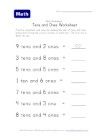Counting Tens and Ones Worksheet

Each question tells kids the number of groups of t...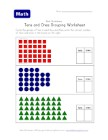Tens and Ones Grouping Worksheet - Two of Two

Circle the group(s) of tens that you can and then ...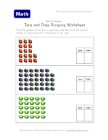Tens and Ones Grouping Worksheet - One of Two

Circle each group of ten and then write the number...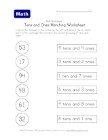Tens and Ones Matching Worksheet - One of Two

Draw a line to match the numbers to the correct gr...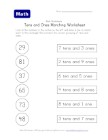Tens and Ones Matching Worksheet - Two of Two

This place value worksheet asks kids to match the ...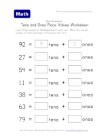Tens and Ones Place Value Worksheet - Two of Two

Break the total number down into its place values ...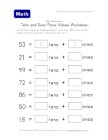Tens and Ones Place Value Worksheet - One of Two

Learn place values by filling in the boxes with th...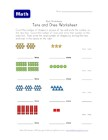Counting Shapes in Tens and Ones

Count the groups and tens and ones - then write th...